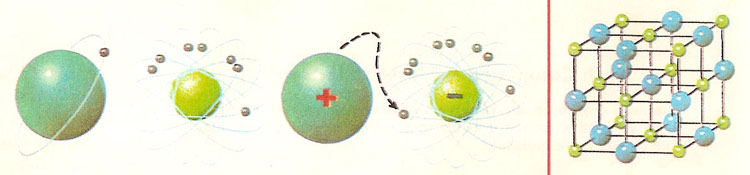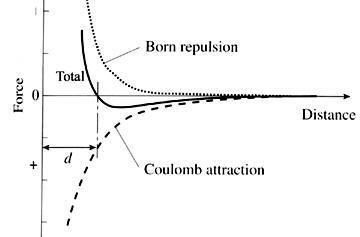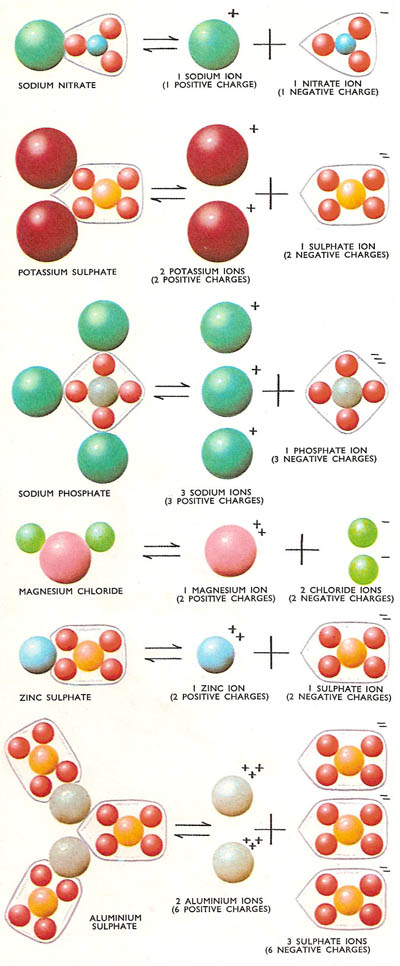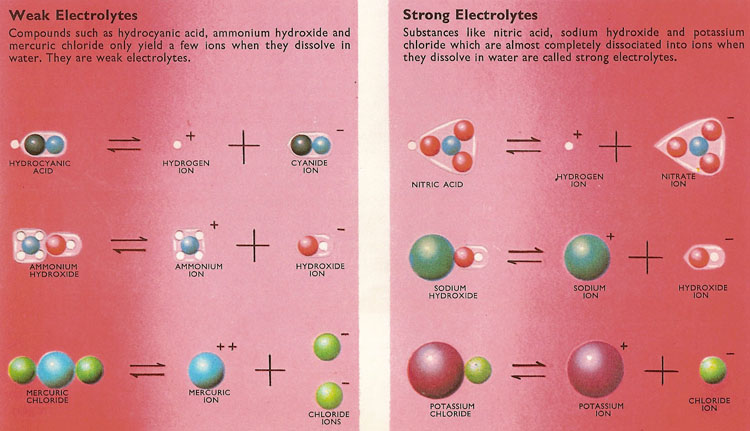A# ionFigure 1. (Left) In the formation of sodium chloride from metallic sodium and gaseous chlorine, the sodium atom donates one electron to the chlorine atom. In this way, a positively charged sodium ion and a negatively charged chlorine ion are formed. The outer electron shells of both ions have been filled. (Right) Model showing position of sodium ions and chloride ions in a crystal of common salt.Figure 2. Ionic radius.Figure 3. Some common salts and their corresponding ions. Note that each compound is electrically neutral, as is the combination of their ions.Figure 4. Weak and strong electrolytes.

An ion is any atom or group of atoms that has become electrically charged by the gain or loss of (negatively-charged) electrons. In general, ions formed from metals are positive (cations), while those from nonmetals are negative (anions). Compound anions are usually anions derived from acids which contain oxygen (oxoacids), e.g., the sulfate ion (SO42-).

### Ionic crystal

An ionic crystal is a type of crystal in which the component ions are held in their positions in the lattice by ionic bonds. Sodium chloride is a typical example. Crystals of ionic compounds consist of negative and positive ions arranged alternately in a lattice and held together by electrical attraction.

### Ionization

Ionization is the formation of ions by the removal or addition of one or more electrons from the outer parts of atoms.

### Ionic bond

An ionic bond is the strong bond that acts in a substance between neighboring ions (see Figure 1).

## Characteristics of ions

### Charge number

Charge number is a dimensionless positive or negative integer which determines the net charge of an ion. Its value is equal to the number of electrons which must be gained or lost by an ion in order achieve a stable state according to the octet rule.

The charge of an ion is the product of the specific charge number and the elementary charge.

The ionic radius is the radius of an atom in its ionized state. Ionic radii depend on two factors: the degree of ionization and the type and number of neighboring atoms (coordination). The radius decreases as electrons are lost and increases as electrons are gained. The length of an ionic bond reflects a balance between the electrostatic Coulomb attraction of the unlike charges and the mutual Born repulsion of the positively charged nuclei (see Figure 2).

The effect of surrounding a cation with anions is to expand its radius. For example, a sodium ion (Na+) with four neighboring oxygen atoms has a radius of 99 picometers (pm), but when surrounded by 12 oxygen atoms its radius is 139 pm.

Cation radii are derived by measuring the bond length (d) and subtracting the anion radius (R-).

## Ions formed in solution

When an acid, a base, or a salt is dissolved in water, some, if not, all of the molecules dissociate (break up) into ions. The metallic ions and hydrogen are positively charged whole the acid radicals and hydroxyl ions are negatively charged.

Although positively and negatively charged ions are set free as a result of ionization, the solution as a whole is electrically neutral. This is because the total charges on the positive and negative ions set free by each molecule of the compound are the same. For example, when a molecule of potassium sulfate ionizes, three ions are produced – two potassium ions each carrying a single positive charge and one sulfate ion carrying two negative charges. This makes a total for each molecule of two positive charges and two negative charges.

The charge carried by an ion is equal to the valence (or valency) of the corresponding atom. This is no coincidence since the ions which make up the molecules are held together by the electrical attraction between unlike charges. It is for this reason that the compounds which ionize in solution are said to be electrovalent. Thus a zinc ion has two charges and has a valence of two. In electrovalent compounds one zinc ion will be associated with a single ion carrying two negative charges (e.g., a sulfate ion) or two ions carrying single negative charges (e.g., two chloride ions).

## How atoms become ions

At the center of each atom is a heavy nucleus containing neutral neutrons and positively-charged protons. Orbiting around the nucleus are a number of lightweight, negatively charged electrons. The number of electrons in any atom is normally equal to the number of protons, so the atom as a whole is electrically neutral.

The orbiting electrons are arranged in shells and it is found that if there are eight electrons in the outer shell (or two in the case of helium) then the atom is unreactive. All atoms try to achieve this most stable arrangement, and to do so they may either gain or lose electrons in the outer shell. These electrons may be gained from, or given to, the atom of another element which also becomes stable. In this way ions are formed from atoms.

Thus an atom of sodium has one electron in its outer electron shell. It readily loses this one electron and so becomes a positively charged ion – there are 11 protons in the nucleus, but now only 10 electrons orbiting it. In contrast, there are seven electrons in the outer shell of chlorine atom, so that by gaining an electron a stable chloride ion is formed. There are now 18 electrons orbiting the chloride ion, but only 17 protons in its nucleus so it has a net single negative charge.

It should be noted that the chemical properties of the atoms and the corresponding ions show marked differences. Both sodium and chlorine are very reactive elements – sodium metal reacts violently with water and hydrogen gas is set free, while chlorine gas has a powerful bleaching action. However, sodium chloride (common salt) has neither of these properties although it contains only sodium and chlorine, but the compound even in its crystalline state is made up of sodium ions and chlorine ions (see Figure 3).

## Electrolytes and electrolysis

Substances such as acids, bases, and salts, which ionize when they dissolve in water are called electrolytes (see Figure 4). An electric current will flow through such a solution, although water itself is a very poor conductor of electricity. The flow of electrons which constitutes an electric current can only move around a completed circuit. When the current passes along wires, there is a movement of free electrons through the metal. However, in an electrolyte the current is able to pass because the electrically charged ions migrate to the electrode of opposite charge under the influence of the electrical pressure (voltage). The process of decomposing an electrovalent compounds is known as electrolysis, and is the basis for example of electroplating.

## Strong and weak acids

Acids such as nitric acid and bases such as sodium hydroxide sodium are often said to be strong. Closer examination of these and other strong electrolytes reveals that the substances are almost completely ionized in solution. In general, a salt (e.g., potassium chloride) obtained by neutralizing a strong acid with a strong base will also ionize almost completely in solution.

At the other end of the range there are weak acids such as hydrocyanic (prussic) acid and weak bases like ammonia. It is found that these compounds are only slightly ionized in solution. The number of ions in a given volume of the solution of a particular weak electrolyte does not vary very much for quite large changes in the concentration of the electrolyte.

## Ionization by collision and bombardment

Ions are also created when atoms or molecules, for example, in a gas, are struck by high-energy particles such as X-rays or fast-moving electrons. The effect is particularly noticeable with gases at low pressures, which sometimes give out colored light when their molecules become ionized. Aurora are the result of gas molecules, especially those of oxygen and nitrogen, being bombarded at high altitudes by fast-moving charged particles from the Sun.